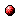# Fuzzy approximation for convergent model-based reinforcement learning

Reference:
L. Busoniu, D. Ernst, B. De Schutter, and R. Babuska, "Fuzzy approximation for convergent model-based reinforcement learning," Proceedings of the 2007 IEEE International Conference on Fuzzy Systems (FUZZ-IEEE 2007), London, UK, pp. 968-973, July 2007.

Abstract:
Reinforcement Learning (RL) is a learning control paradigm that provides well-understood algorithms with good convergence and consistency properties. Unfortunately, these algorithms require that process states and control actions take only discrete values. Approximate solutions using fuzzy representations have been proposed in the literature for the case when the states and possibly the actions are continuous. However, the link between these mainly heuristic solutions and the larger body of work on approximate RL, including convergence results, has not been made explicit. In this paper, we propose a fuzzy approximation structure for the Q-value iteration algorithm, and show that the resulting algorithm is convergent. The proof is based on an extension of previous results in approximate RL. We then propose a modified, serial version of the algorithm that is guaranteed to converge at least as fast as the original algorithm. An illustrative simulation example is also provided.Corresponding technical report: pdf file (166 KB)
Note: More information on the pdf file format mentioned above can be found here.

Bibtex entry:

@inproceedings{BusErn:07-011,
author={L. Bu{\c{s}}oniu and D. Ernst and B. {D}e Schutter and R. Babu{\v{s}}ka},
title={Fuzzy approximation for convergent model-based reinforcement learning},
booktitle={Proceedings of the 2007 IEEE International Conference on Fuzzy Systems (FUZZ-IEEE 2007)},